# 5 MUST KNOW VEDIC MATH TRICKS FOR YOUR KID

Vedic Mathematics is derived from a Sanskrit word "Veda" which means "Knowledge". It is a collection of techniques/sutras to solve mathematical problems in a faster and easy way. It has 16 Sutras ( Formulae) and 13 sub-sutras ( Sub Formulae). These sutras help to solve any problem related to arithmetic, algebra, geometry, calculus, conics. It is considered that by using Vedic Math tricks one can solve math problems 10 to 15  times faster than any other usual method.

These tricks are faster and easier and if done in their proper manner give accurate answers. They are very helpful for kids in their regular school work calculations and also while cracking exams like CAT, CET, SAT, Banking Exams, etc.

TRICK NO:1  Multiplying numbers by 5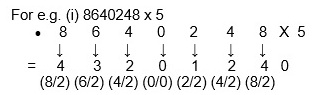Therefore we get, 8640248 x 5 = 43201240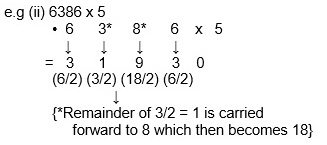Therefore we get, 6386 x 5 = 31930

Simply, consider the given number to be ‘A’

Therefore,          A X 5 = A X 10/2

which is again  = A/2 X 10

TRICK NO:2   Multiplying any number by 11

For e.g.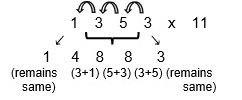Step I : Last digit remains same.

Step II : Add every digit starting from end to the previous one, move from right to left.

Step III : First digit remains same.

Therefore we get, 1353 x 11 = 14883

TRICK NO:3  [T20:20]  * Applied when both the numbers are more than 10 and less than 20.

For e.g.           13 x 16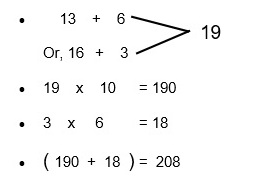Step I : Sum of first number and last digit of second number or, last digit of first number and second number.

Step II : Answer of Step I x 10

Step III : Multiply last digit of both the numbers.

Step IV : Add the solutions of Step II and Step III.

Therefore we get, 13  x  16  = 208.

TRICK NO:4  [X + T10]  *  Applicable when sum of last digit of both the numbers gives 10, and first digit of both the numbers are same.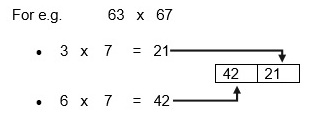Step I: Multiply last digit of both the numbers.

Step II: Multiply the first digit of both the numbers with the digit that comes after that i.e., 7 comes after 6 so 6x7 in the above case.

Therefore we get, 63 x 67 = 4221.

TRICK NO:5  Multiplying numbers by 12.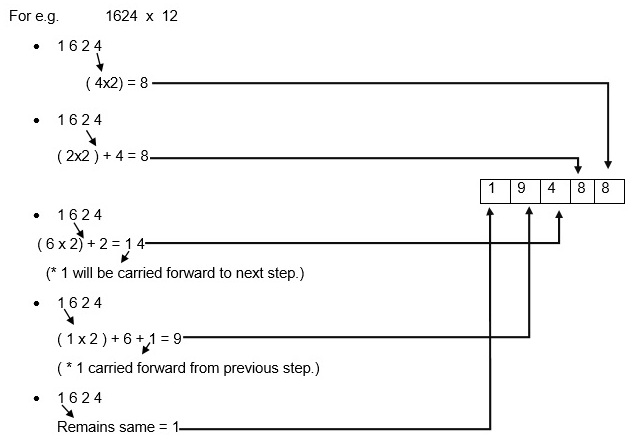Step I: Last digit will be double.

Step II: Multiply the previous digit with 2 and add it with the next digit. If there is any previous remainder add it with that.

Step III: First digit of the number is reused and kept same in the last step.

Therefore, we get, 1624 x 12 = 19488.

These were few simple Vedic Math tricks that make calculation faster and easier. These tricks are far more systematic, simplified and unified than the conventional system and thus give accurate answers.  The fact still remains that not even 20% of schools today, teach students the tricks of Vedic math. Although you might find a few online lessons or after school classes like Speed Maths, (an Aloha initiative) to impart the knowledge of this amazing age old methodology of arithmetic. Application of these tricks gives the student a lot of flexibility, fun and satisfaction. It not only helps them to save their precious time in examination hall, but also acts as a checking tool. The only key is a regular practice and application in all questions they come across. Once you teach a trick to your child just make sure he/she applies it everywhere possible. You will see wonders happening soon!

To Top ↑